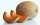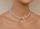# Real numbers + addition - math problems

#### Number of problems found: 8

• ChoseChose Juan bought three pieces of watermelon. If the weights of the three watermelons are 2.2103 kilograms, 3.0214 kilograms, and 2.6682 kilograms how many kilograms of watermelons did Chose Juan buy?
• ItemsItems Acosts 675.35 while item B costs 1605.25. by how much more was the cost of item B than item A. If item A and B will be purchased, how much would are pay?
• The sum 10The sum of the three decimals is 938.629. Two of them are 456.54 and 392.69. Find the third one.
• Josh worksJosh works mowing lawns and babysitting. He earns \$7.20 an hour for mowing and \$6.80  an hour for babysitting. How much will he earn for 1 our of mowing and 6 hours of babysitting?
• NecklacesMohammad sells beaded necklaces. Each large necklace sells for \$4.50 and each small necklace sells for \$4.10. How much will he earn from selling 5 large necklaces and 4 small necklaces?
• ZOO 2Valerie bought tickets to the zoo for the family. She bought three adult tickets for \$9.50 each and two children's tickets for \$4.50 each. How much did the tickets cost Valerie in all?
• Imaginary numbersFind two imaginary numbers whose sum is a real number. How are the two imaginary numbers related? What is its sum?
• Short cutImagine that you are going to a friend. That path has a length 120 meters. Then turn doprava and go other 630 meters and you are at a friend's. The question is how much the journey will be shorter if you go direct across the field?

We apologize, but in this category are not a lot of examples.
Do you have an exciting math question or word problem that you can't solve? Ask a question or post a math problem, and we can try to solve it.

We will send a solution to your e-mail address. Solved examples are also published here. Please enter the e-mail correctly and check whether you don't have a full mailbox.

Real numbers - math problems. Addition Problems.